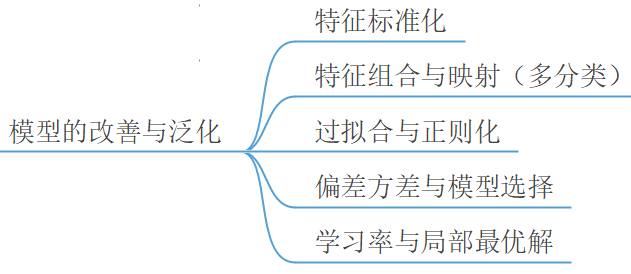### 4.2特征标准化

#### 4.2.1等高线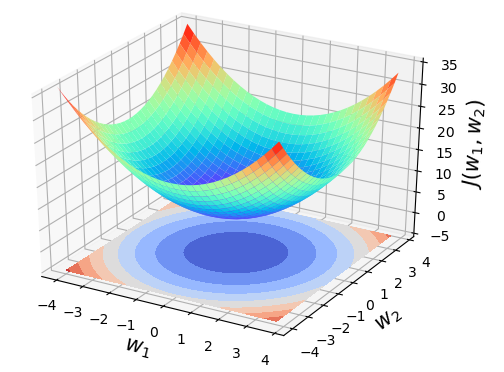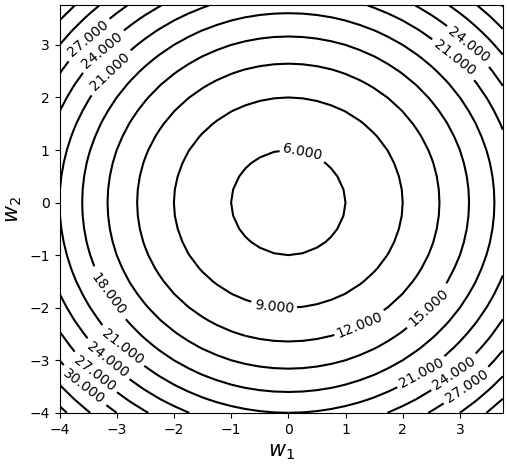#### 4.2.2梯度与等高线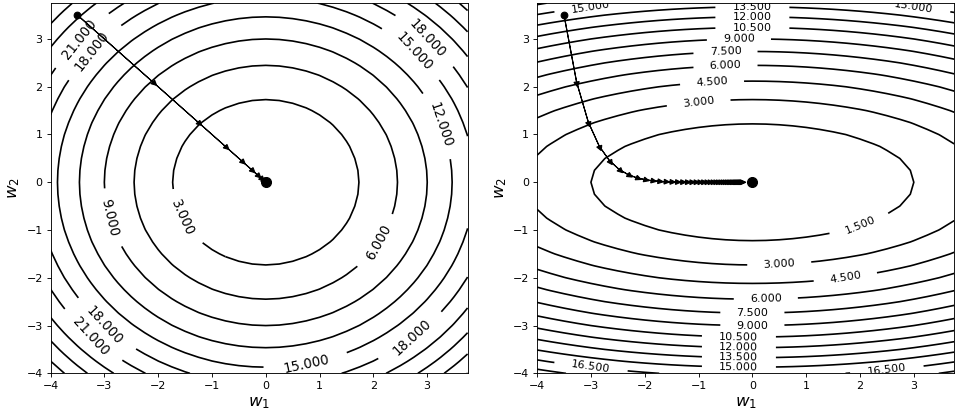$f(x,y)=c$为平面上任意曲线，又由于曲线$F(x,y)=f(x,y)-c=0$的法向量为$\overrightarrow{n}=\{F_x,F_y\}=\Delta F$。故，曲线$F(x,y)$的法向量为$\vec{m}=\{{{f}_{x}},{{f}_{y}}\}$。可以发现，曲线$F(x,y)$也就是$f(x,y)=c$的法向量$\vec{m}$正好就是曲线$f(x,y)=c$对应的梯度，所以可以得出梯度垂直于曲线（等高线）的结论。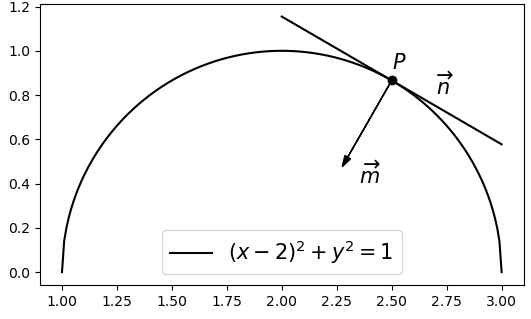$y=\sqrt{1-{{(x-2)}^{2}}}$代入$(4.1)$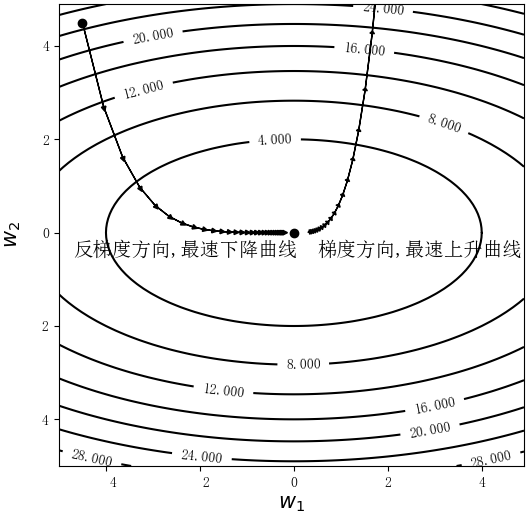#### 4.2.3标准化方法

1) 线性回归

2) 逻辑回归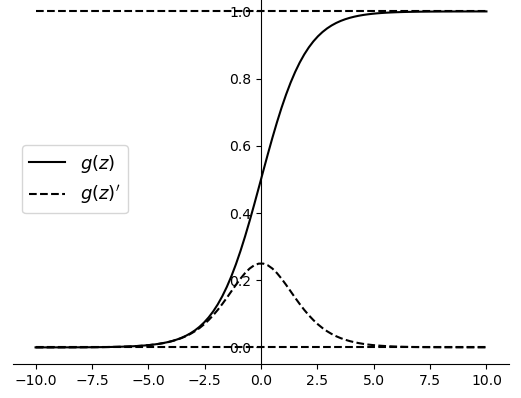xxxxxxxxxx41def standarlization(X):2    mean = X.mean(axis=0)3    std = X.std(axis=0)4    return (X - mean) / std

#### 4.2.4 特征组合与映射

2.3.1节中介绍线性回归时，笔者通过一个预测梯形面积的示例来解释了特征组合的作用（丰富特征属性以提高预测精度）。但其实还可以从另外一个角度来理解特征组合。线性回归和逻辑回归从本质上讲都属于线性模型，因此对于基本的线性回归模型和逻辑回归模型来讲，其分别只能用于预测线性变化的实数和线性可分的类别，即对于如下图4-8所示的两种任务，两个基本模型均不能够完成。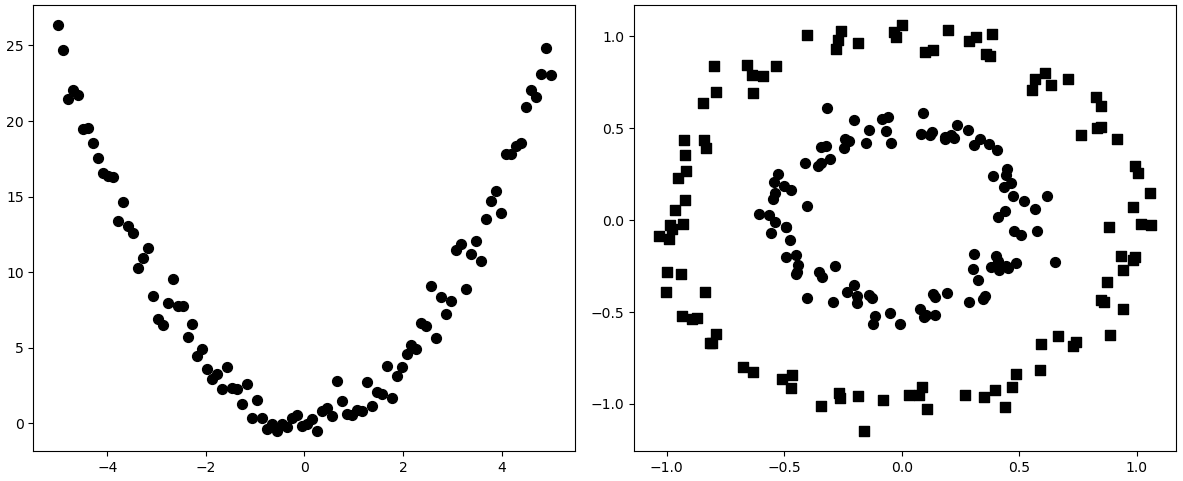1) 非线性回归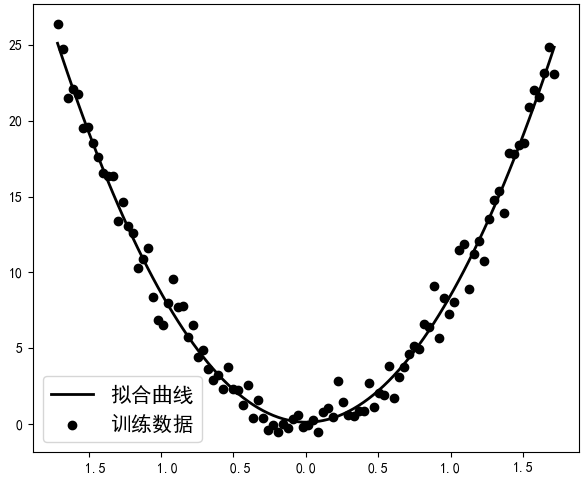2) 非线性分类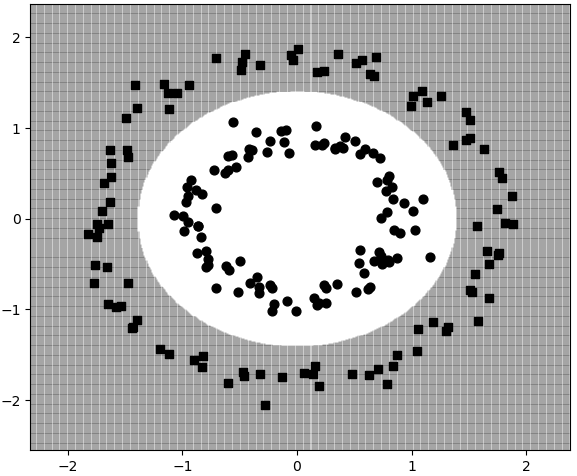### 4.3 过拟合

#### 4.3.1 模型拟合

2.5节内容中，笔者首次引入了梯度下降算法来最小化线性回归中的目标函数，并且在经过多次迭代后便可以求得到模型中对应的参数。此时可以发现，模型的参数是一步一步根据梯度下降算法更新而来直至目标函数收敛，也就是说这是一个循序渐进的过程。因此，这一过程也被称作是拟合（Fitting）模型参数的过程，当这个过程执行结束后就会产生多种拟合后的状态，例如过拟合（Overfitting）和欠拟合（Underfitting）等。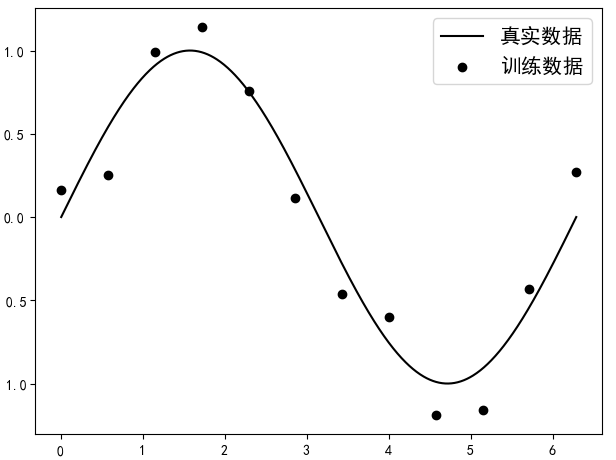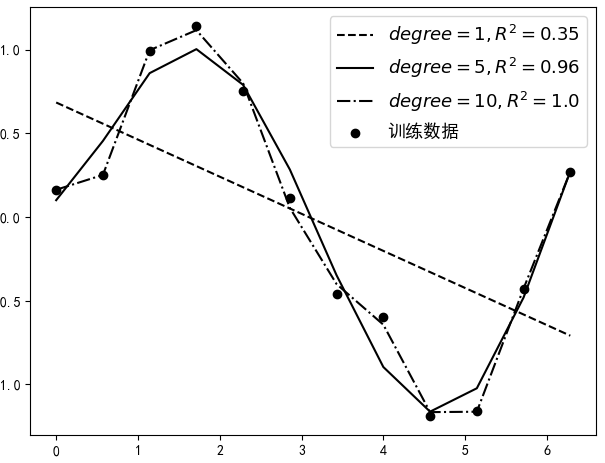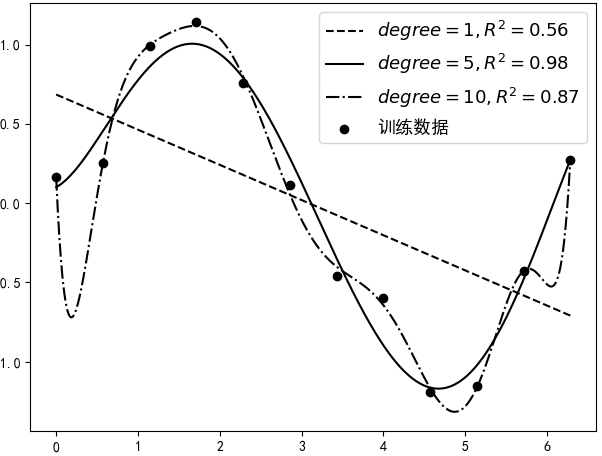#### 4.3.3 解决欠拟合与过拟合

1) 如何解决欠拟合

• 设计新的特征；收集或设计更多的特征维度作为模型的输入，即根据已有特征数据组合设计得到更多新的特征，这有点类似于上一点。
• 减小正则化系数；当模型出现欠拟合现象时，可以通过减小正则化中的惩罚系数来减缓欠拟合现象，这一点将在4.4节中进行介绍。

2) 如何解决过拟合

• 收集更多数据；这是一个最为有效但实际操作起来又是最为困难的一个方法。训练数据越多，在训练过程中也就越能够纠正噪音数据对模型所造成的影响，使得模型不易过拟合。但是对于新数据的收集往往具有较大的困难。
• 降低模型复杂度；当训练数据过少时，使用较为复杂的模型极易产生过拟合现象，例如4.3.1中的示例。因此可以通过适当减少模型的复杂度来达到缓解模型过拟合的现象。
• 正则化方法；在出现过拟合现象的模型中加入正则化约束项，以此来降低模型过拟合的程度，这部分内容将在4.4节中进行介绍。
• 集成方法；将多个模型集成在一起，以此来达到缓解模型过拟合的目的，这部分内容将在第8章中进行介绍。

3) 如何避免过拟合

### 4.4 正则化

#### 4.4.2 训练集导致糟糕的泛化误差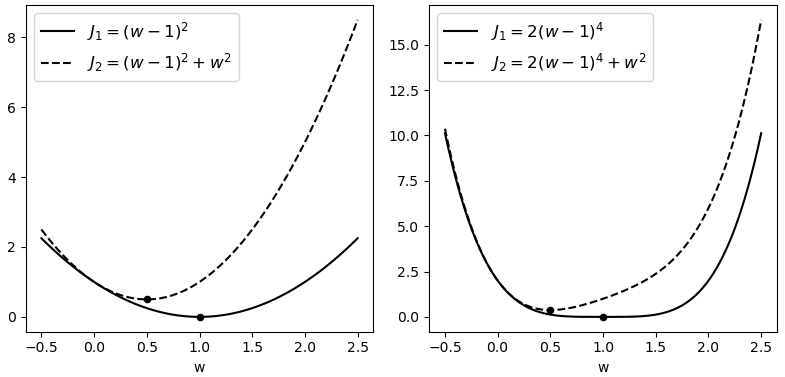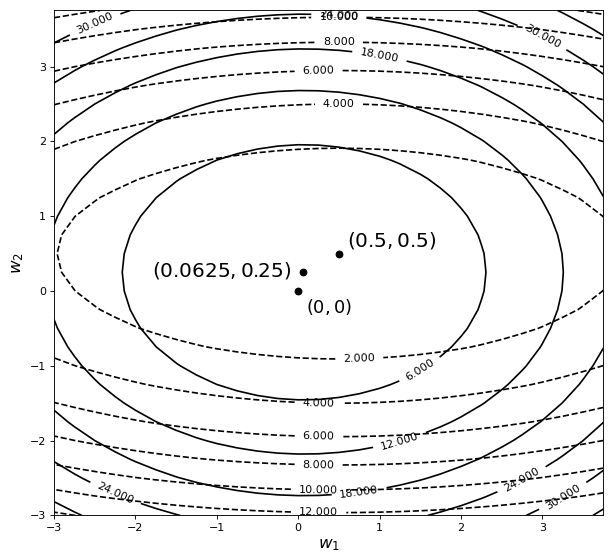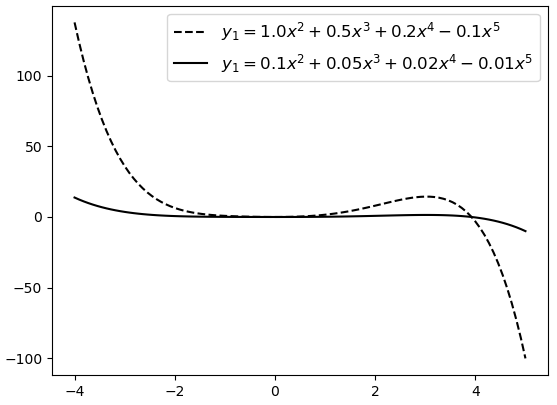1) 线性回归

2) 逻辑回归

#### 4.4.4 正则化示例代码

1) 制作数据集

xxxxxxxxxx71def make_data():2    n_train, n_test, n_features = 50, 100, 1003    w, b = np.ones((n_features, 1)) * 0.2, 2 4    x =np.random.normal(size=(n_train + n_test, n_features))# 5    y = np.matmul(x, w) + b #用来生成正确标签6    y += np.random.normal(scale=0.2, size=y.shape)7    return x,u

2) 定义目标函数

xxxxxxxxxx61def cost_function(X, y, W, bias, lam):2    m, n = X.shape3    y_hat = prediction(X, W, bias)4    J = 0.5 * (1 / m) * np.sum((y - y_hat) ** 2) 5    Reg = lam / (2 * n) * np.sum(W ** 2)         #正则化项6    return J + Reg

3) 定义梯度下降

xxxxxxxxxx81def gradient_descent(X, y, W, bias, alpha, lam):2    m, n = X.shape3    y_hat = prediction(X, W, bias)4    grad_w = -(1 / m) * np.matmul(X.T, (y - y_hat)) + (lam / n) * W 5    grad_b = -(1 / m) * np.sum(y - y_hat) 6    W = W - alpha * grad_w  7    bias = bias - alpha * grad_b8    return W, bias### 4.5 偏差方差与交叉验证

#### 4.5.1 偏差与方差定义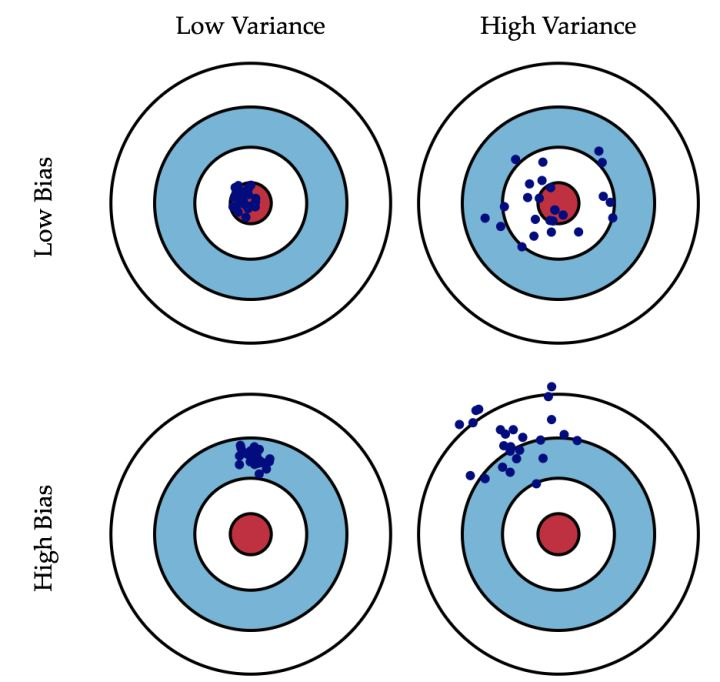• 所有子弹都密集打在靶心旁边的位置，这就是典型的方差小（子弹很集中），偏差大（距离靶心甚远）；
• 子弹都散落在靶心周围的位置，这就是典型的方差大（子弹很散乱），偏差小（都在靶心附近）；
• 子弹都散落在靶心旁边的位置，这就是典型的方差大（子弹散乱），偏差大（距离靶心甚远）；
• 所有子弹都密集打在了红色靶心的位置，这就是典型的方差小（子弹集中），偏差小（都在靶心位置）；

#### 4.5.2 模型的偏差与方差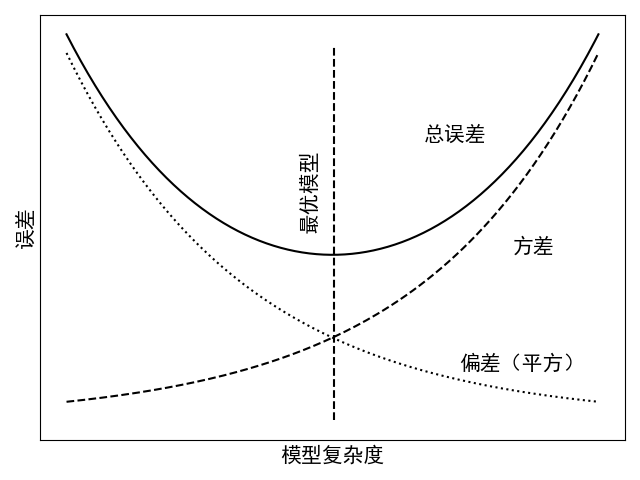#### 4.5.3 超参数选择

1) 惩罚系数$\lambda$

2) 学习率$\alpha$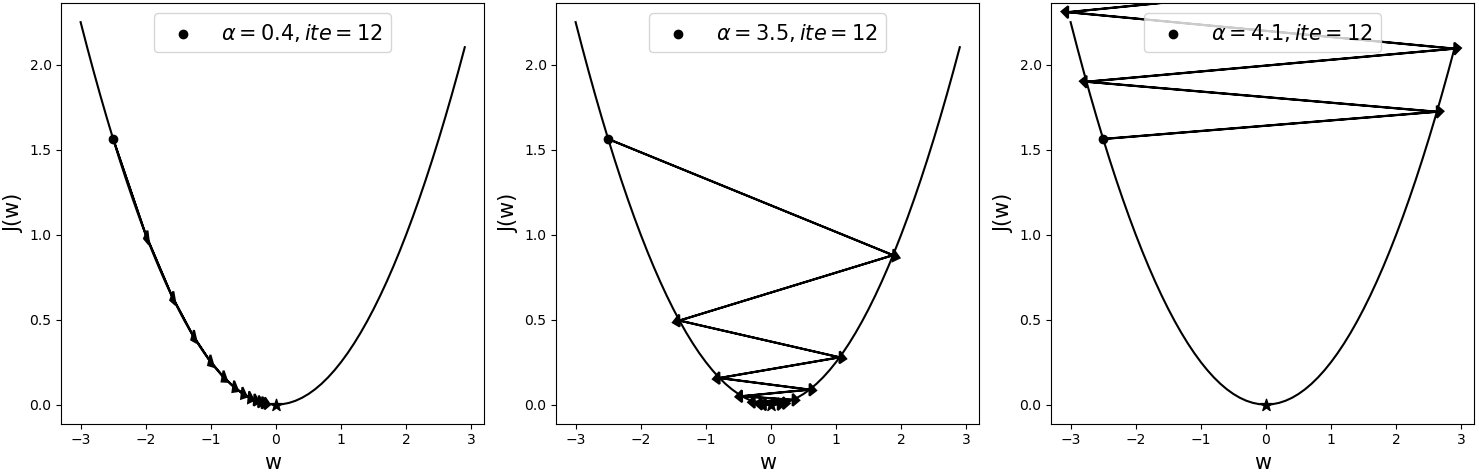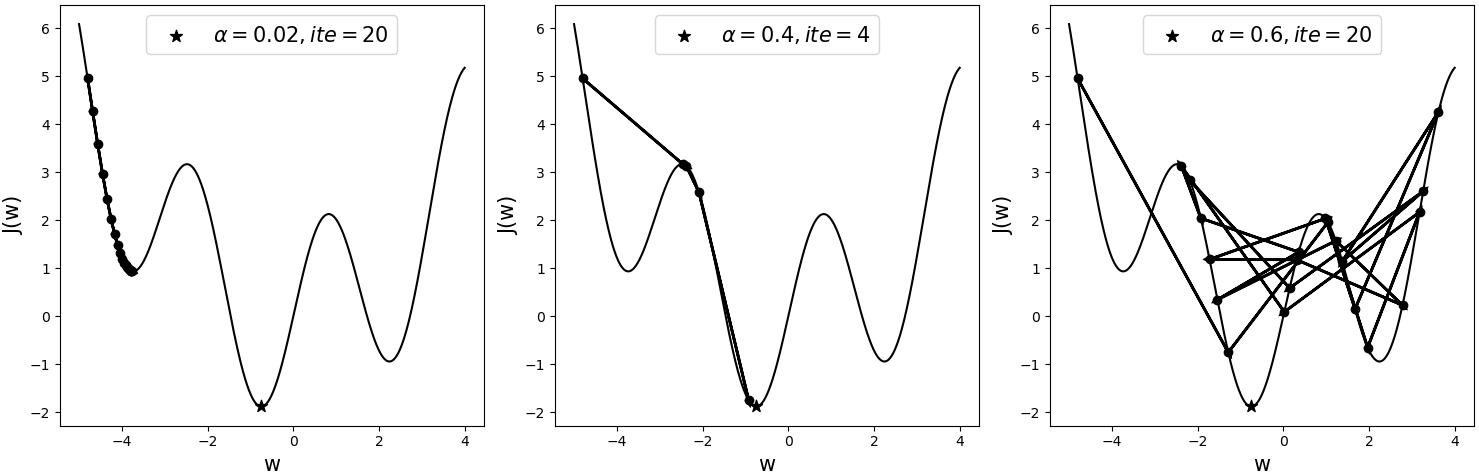#### 4.5.4 模型选择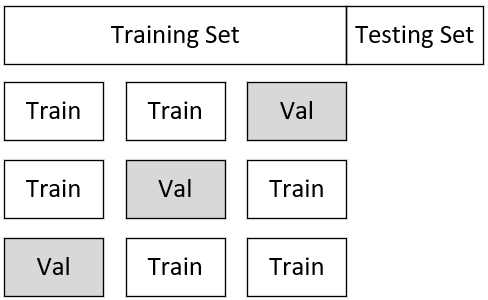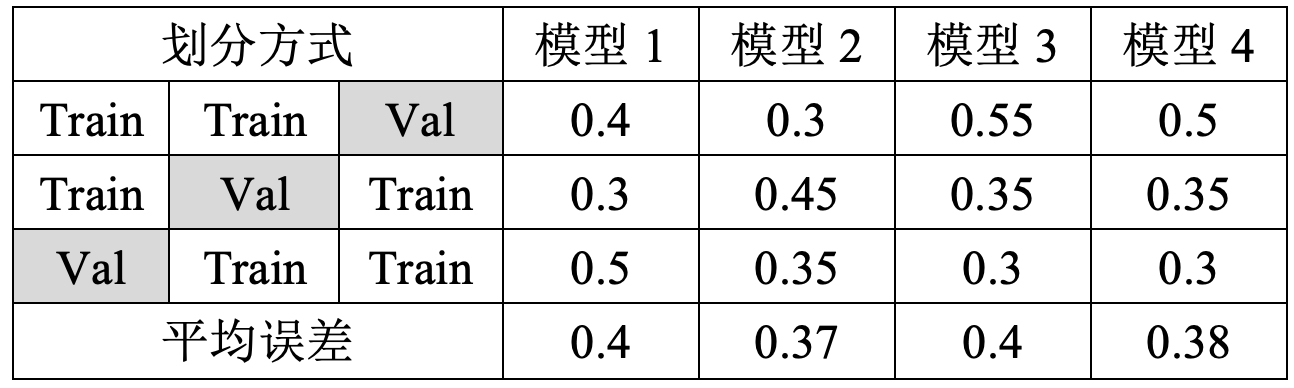### 4.6 实例分析手写体识别

#### 4.6.1 数据预处理

1) 载入数据集

xxxxxxxxxx41def load_data():2    data = load_digits()3    x, y = data.data, data.target4    return x,y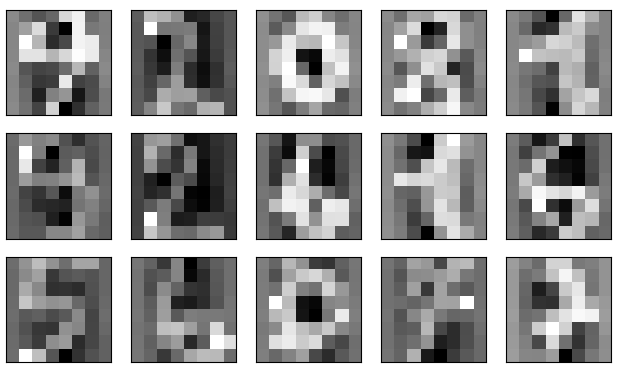2) 划分数据集

xxxxxxxxxx51from sklearn.model_selection import train_test_split2def load_data():3    #此处接“1.载入数据集”中代码4    x_train, x_test, y_train, y_test = \5          train_test_split(x, y, test_size=0.3, random_state=20)

3) 对训练集标准化

xxxxxxxxxx51from sklearn.preprocessing import StandardScaler2def load_data():3    #此处接“2.划分数据集”中代码4    ss = StandardScaler()5    x_train = ss.fit_transform(x_train)

xxxxxxxxxx51def load_data():2    #此处接“2.划分数据集”中代码3    ss = StandardScaler()4    ss.fit(x_train) #先计算每3个维度需要用到的均值和方差 5    x_train = ss.transform(x_train)#再对每个维度进行标准化

4) 对测试集标准化

xxxxxxxxxx31def load_data():2    #此处接“3.对训练集标准化”中代码3    x_test = ss.transform(x_test)

#### 4.6.2 模型选择

1) 列举超参数

xxxxxxxxxx21learning_rates = [0.001, 0.03, 0.01, 0.03, 0.1, 0.3, 1, 3]2penalties = [0, 0.01, 0.03, 0.1, 0.3, 1, 3]

2) 定义模型

xxxxxxxxxx61from sklearn.linear_model import SGDClassifier2for learning_rate in learning_rates:3    for penalty in penalties:4        print(f"训练模型:learning_rate={learning_rate}, penalty={penalty}")5         model = SGDClassifier(loss='log', penalty='l2', learning_rate = 'constant', 6                               eta0=learning_rate, alpha=penalty)

3) 交叉验证

xxxxxxxxxx81from sklearn.model_selection import KFold2model = ……此处接“2.定义模型”中代码3kf = KFold(n_splits=k, shuffle=True, random_state=10)4for train_index, dev_index in kf.split(X):5    X_train, X_dev = X[train_index], X[dev_index]6    y_train, y_dev = y[train_index], y[dev_index]7    model.fit(X_train, y_train)8    s = model.score(X_dev, y_dev)

xxxxxxxxxx51正在训练模型: learning_rate = 0.001, penalty = 02正在训练模型: learning_rate = 0.001, penalty = 0.013……4正在训练模型: learning_rate = 3, penalty = 35最优模型:  [0.9554322392967812, 0.03, 0]

#### 4.6.3 模型测试

xxxxxxxxxx41model = SGDClassifier(loss='log', penalty='l2', learning_rate='constant',                            eta0=0.03, alpha=0.0)2model.fit(x_train, y_train)3y_pred = model.predict(x_test)4print(classification_report(y_test, y_pred))

xxxxxxxxxx41                 precision  recall  f1-score   support2    accuracy                            0.96       5403   macro avg       0.96      0.96       0.96       5404weighted avg       0.96      0.96       0.96       540

### 引用

The Discipline of Machine Learning, Tom M. Mitchell, July 2006 CMU-ML-06-108

Andrew Ng, Machine Learning, Stanford University, CS229, Spring 2019.

Ian，Goodfellow, et al. Deep Learning. 深度学习 中文译本 人民邮电出版社

Hung-yi Lee, Machine Learning, National Taiwan University, 2020, Spring.

李沐, 动手学深度学习, 人民邮电出版社

### 推荐阅读

 第1章 机器学习入门之环境安装配置（附高清PDF）

 第2章 从零认识线性回归（附高清PDF与教学PPT）

第3章 从零认识逻辑回归（附高清PDF与教学PPT）